For a cell, the graph between the potential difference (V) across the terminals of the cell and the current (I) drawn from the cell is shown in the figure. The e.m.f. and the internal resistance of the cell is: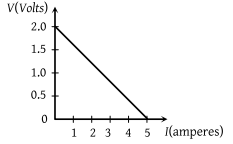(1) 2V, 0.5 Ω

(2) 2 V, 0.4 Ω

(3) > 2 V, 0.5 Ω

(4) > 2 V, 0.4 Ω

Concept Questions :-

Emf and terminal voltage
High Yielding Test Series + Question Bank - NEET 2020

Difficulty Level:

When a current I is passed through a wire of constant resistance, it produces a potential difference V across its ends. The graph drawn between log I and log V will be

(1)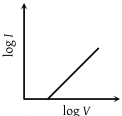(2)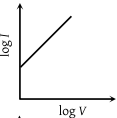(3)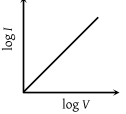(4)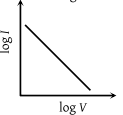Concept Questions :-

Derivation of Ohm's law
High Yielding Test Series + Question Bank - NEET 2020

Difficulty Level:

The V-I graph for a conductor at temperature T1 and T2 are as shown in the figure. $\left({T}_{2}-{T}_{1}\right)$ is proportional to :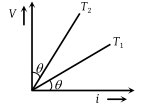(1) $\mathrm{cos}2\theta$

(2) $\mathrm{sin}\theta$

(3) $\mathrm{cot}2\theta$

(4) $\mathrm{tan}\theta$

Concept Questions :-

Current and current density
High Yielding Test Series + Question Bank - NEET 2020

Difficulty Level:

A cylindrical conductor has a uniform cross-section. The resistivity of its material increases linearly from the left end to the right end. If a constant current is flowing through it and at a section distance x from the left end, the magnitude of electric field intensity is E, which of the following graphs is correct :

(1)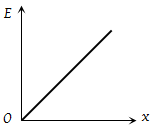(2)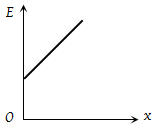(3)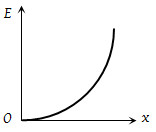(4)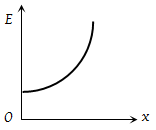Concept Questions :-

Derivation of Ohm's law
High Yielding Test Series + Question Bank - NEET 2020

Difficulty Level:

The V-I graph for a conductor makes an angle θ with V-axis. Here V denotes the voltage and I denotes current. The resistance of the conductor is given by :

(1) $\mathrm{sin}\theta$

(2) $\mathrm{cos}\theta$

(3) $\mathrm{tan}\theta$

(4) $\mathrm{cot}\theta$

Concept Questions :-

Derivation of Ohm's law
High Yielding Test Series + Question Bank - NEET 2020

Difficulty Level:

A battery consists of a variable number 'n' of identical cells having internal resistances connected in series. The terminals of battery are short circuited and the current i is measured. Which of the graph below shows the relation ship between i and n

(1)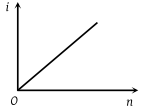(2)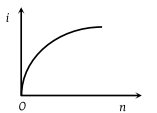(3)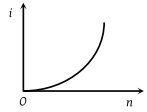(4)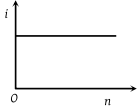Concept Questions :-

Grouping of cells
High Yielding Test Series + Question Bank - NEET 2020

Difficulty Level:

In an experiment, a graph was plotted of the potential difference V between the terminals of a cell against the circuit current I by varying load rheostat. Internal conductance of the cell is given by :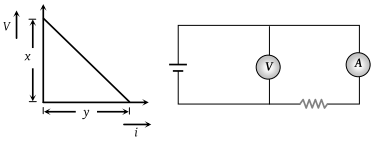(1) xy

(2) $\frac{y}{x}$

(3) $\frac{x}{y}$

(4) (xy)

Concept Questions :-

Emf and terminal voltage
High Yielding Test Series + Question Bank - NEET 2020

Difficulty Level:

V-i graphs for parallel and series combination of two identical resistors are as shown in the figure. Which graph represents a parallel combination ?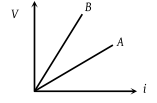1. A

2. B

3. A and B both

4. Neither A nor B

Concept Questions :-

Combination of resistors
High Yielding Test Series + Question Bank - NEET 2020

Difficulty Level:

The ammeter has range 1 ampere without shunt. the range can be varied by using different shunt resistances. The graph between shunt resistance and range will have the nature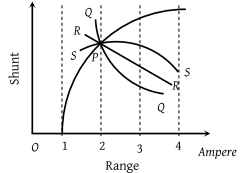(1) P

(2) Q

(3) R

(4) S

High Yielding Test Series + Question Bank - NEET 2020

Difficulty Level:

Resistance as shown in figure is negative at(1) A

(2) B

(3) C

(4) None of these

Concept Questions :-

Derivation of Ohm's law